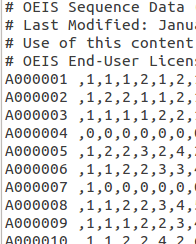To show that interpreted languages can be fast when used well, I’m posting this example.

Take the On-line Encyclopedia of Integer Sequences database, which is a collection of integer sequences. That means lists of numbers. You can get a file from http://oeis.org/stripped.gz, which contains the first few numbers of each sequence. Today’s file extracts to about 38MB.

Now I need to do lookups in this file for a program I’m writing, and that program is in PHP. We want to know some sequences based on their A-number, like this:

``````\$primes = oeis("A000040");
\$fibonacci = oeis("A000045");
``````

If we’re smart about it, then even a large file can be parsed in fractions of a second. Here’s how we do it:

``````/* This code needs an extract of the OEIS database to operate.
I got it from http://oeis.org/stripped.gz
Just extract that to this folder for lookups */
function oeis(\$number) {
/* Return an array of values based on a sequence's OEIS number */
\$number = strtoupper(\$number);
\$fp = fopen("stripped", "r");
while(\$ln = fgets(\$fp)) { /* Find this sequence and break the loop */
if(substr(\$ln, 0, strlen(\$number) + 1) == \$number." ") {
\$res = \$ln;
break;
}
}
fclose(\$fp);
/* Exit if we haven't got anything */
if(!isset(\$res)) { return false; }
\$rv = explode(" ", \$ln); /* Split lines into left and right of space */
\$ln = trim(\$rv);
\$ln = substr(\$ln, 1, strlen(\$ln)-2); /* Slices off extra commas on sides */
\$rv = explode(",", \$ln); /* Split by commas */
return \$rv;
}``````

Note that we don’t use explode() until after we have found the line we need, and also note that file_get_contents() is not used at all. (Multi-megabyte strings will bog you down in any language).